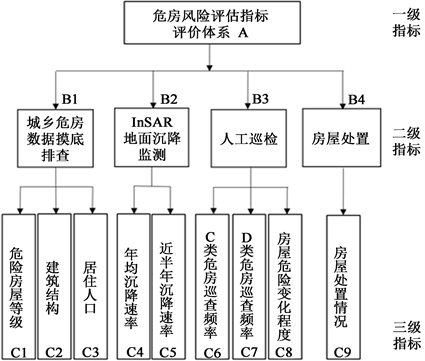#### 期刊菜单

Comprehensive Evaluation Method of Urban Dangerous Building Safety Based on Analytic Hierarchy Process
DOI: 10.12677/HJCE.2022.116086, PDF, HTML, XML, 下载: 67  浏览: 111

Abstract: The comprehensive safety evaluation of urban dangerous buildings is an important work to ensure the safety of housing for the national poverty alleviation campaign. The conditions of urban buildings have deteriorated to various degrees due to the long construction year and the influence of natural disasters. However, the time, equipment and labor costs to conduct extensive building safety evaluation with a variety of testing equipment are expensive. This paper proposes the use of the analytic hierarchy process, combined with the relevant national standards, to evaluate the safety conditions of urban dangerous buildings, which can be the basis for building safety management and decision-making.

1. 引言

2. 基于层次分析法的城市危险房屋安全综合评价基本原理

2.1. 层次分析法

2.1.1. 构建评价指标体系，建立层次结构模型

2.1.2. 同一准则层间各元素两两比较，依据其相对重要性构建判断矩阵Table 1. 1~9 Scale method meaning table

2.1.3. 层次单排序及一致性检验

${w}_{i}=\frac{{M}_{i}}{{\sum }_{i=1}^{n}{M}_{i}}=\frac{\sqrt[n]{{\Pi }_{j=1}^{n}{a}_{ij}}}{{\sum }_{i=1}^{n}{M}_{i}}$ (1)

$CR=\frac{CI}{RI}$ (2)

${\lambda }_{\mathrm{max}}=\underset{i=1}{\overset{n}{\sum }}\frac{{\sum }_{i=1}^{n}{a}_{ij}{w}_{i}}{n{w}_{i}}$ (3)

$RI$ 是判断矩阵的平均随机一致性指标。对于1~10阶判断矩阵， $RI$ 的取值参照下表2所示。

2.1.4. 层次总排序及一致性检验Table 2. Mean random consistency index

2.1.5. 综合评价

${A}_{危房}={\sum }_{i=1}^{n}\left({a}_{i}\cdot {t}_{i}\right)$ (4)Table 3. Unsafe building safety assessment risk index

3. 计算实例Table 4. Urban dangerous building scene index and definitionsFigure 1. Evaluation system of unsafe house safety risk assessment index

$\left\{\begin{array}{ll}{A}_{危房}=100\hfill & {c}_{1}\text{or}{c}_{3}=100\hfill \\ {A}_{危房}={\sum }_{i=1}^{n}\left({c}_{i}\cdot {t}_{i}\right)\hfill & {c}_{1}\text{and}{c}_{3}\ne 100\hfill \end{array}$ (5)Table 5. Evaluation system of unsafe house safety risk assessment indexTable 6. A-B weight vector calculation and consistency testTable 7. B1-C weight vector calculation and consistency testTable 8. B2-C weight vector calculation and consistency testTable 9. B3-C weight vector calculation and consistency test

4. 结论

  浙江省建筑科学设计研究院有限公司. 浙江省地质灾害危险性评估规范(DB33T881-2012) [S]. 北京: 标准出版社, 2013.  重庆市土地房屋管理局, 上海市房地产科学研究院. 危险房屋鉴定标准(JGJ 125-2016) [S]. 北京: 标准出版社, 1999.  杨修歌. 模糊层次分析法在村镇房屋鉴定中的应用[J]. 盐城工学院学报(自然科学版), 2019, 32(4): 59-62.  李胜波, 王建国, 谢会川. 运用模糊数学结合层次分析法评价房屋完损等级[J]. 四川建筑科学研究, 2004(1): 66-68.  杜运兴, 赵光超, 周芬. 区域住宅安全状况综合评价方法研究[J]. 自然灾害学报, 2013, 22(3): 185-191.  杜运兴, 赵光超, 周芬. 区域住宅安全等级评价方法研究[J]. 湖南大学学报(自然科学版), 2012, 39(1): 21-26.  范丽远. 层次分析法在城市街区防震减灾能力评价中的应用研究[D]: [硕士学位论文]. 吉林: 吉林大学经济学系, 2015.  张磊. 基于模糊层次分析法的印制板厂安全预评价研究[D]: [硕士学位论文]. 四川: 西南科技大学资源与环境系, 2018.